﻿ Theoretical-Experimental Study of the Effect of the External Plates on the Thermal Performance of a Plate Heat Exchanger

### Theoretical-Experimental Study of the Effect of the External Plates on the Thermal Performance of a ...

Julio del Angel Soto, Martín T. Martínez G., José Luis Magaña

Chemical Engineering and Science

## Theoretical-Experimental Study of the Effect of the External Plates on the Thermal Performance of a Plate Heat Exchanger

Julio del Angel Soto1, Martín T. Martínez G.1, José Luis Magaña1,1Department of Chemicals Engineering, University of Guanajuato, Cerro de la venada s/n, CP. 36040

### Abstract

In this work we present a theoretical-experimental research on the thermal phenomena that the endplates present on the performance of the plate heat exchangers in a single step and with a U type configuration. The endplate effect is reflected in a reduction of the temperature difference for heat transfer. The model discusses a theoretical focus that includes the elongation factor, which is a parameter that relates the actual area to the projected area of a plate. This model was performed to 2, 3, 4, 5, and 6 channel flow; therefore the experimentation was carried out to validate the theoretical model, it was carried out for 4, 5, 6, 7, 8 and 9 flow channels. Such results show that the theoretical model deviates in a 5% of the experimental values. The results obtained for theorical temperature correction factor presents a maximum deviation of ± 0.0245 with respect to the experimental values.

### Cite this article:

• Julio del Angel Soto, Martín T. Martínez G., José Luis Magaña. Theoretical-Experimental Study of the Effect of the External Plates on the Thermal Performance of a Plate Heat Exchanger. Chemical Engineering and Science. Vol. 3, No. 1, 2015, pp 12-18. http://pubs.sciepub.com/ces/3/1/3
• Soto, Julio del Angel, Martín T. Martínez G., and José Luis Magaña. "Theoretical-Experimental Study of the Effect of the External Plates on the Thermal Performance of a Plate Heat Exchanger." Chemical Engineering and Science 3.1 (2015): 12-18.
• Soto, J. D. A. , G., M. T. M. , & Magaña, J. L. (2015). Theoretical-Experimental Study of the Effect of the External Plates on the Thermal Performance of a Plate Heat Exchanger. Chemical Engineering and Science, 3(1), 12-18.
• Soto, Julio del Angel, Martín T. Martínez G., and José Luis Magaña. "Theoretical-Experimental Study of the Effect of the External Plates on the Thermal Performance of a Plate Heat Exchanger." Chemical Engineering and Science 3, no. 1 (2015): 12-18.

 Import into BibTeX Import into EndNote Import into RefMan Import into RefWorks

1234
Prev Next

### 1. Introduction

The plate heat exchangers (PHE) have been widely used in food processing, chemical industrial processes, and other industrial applications for many years. The advantages of using them have been clearly indicated in the work reported by [1, 2, 3, 4], among them can be found the requirement of low physical space for its location on the ground, higher thermal efficiencies, low tendency to fouling, high flexibility of the arrangement of streams and easy maintenance. PHE design involves determining the optimal pressure drops, minimizing heat transfer area, minimizing capital costs and operational costs, holding a balance between these factors, which depend on the limitations of each case [5, 6, 7, 8].

The most commercial plate used in the design of a PHE is the chevron or herringbone type, Figure 1. This design is based on a corrugation angle β (called chevron angle) for the liquid flow, which combined, at different angles or opposite, makes contact at several points allowing the flow between them and generating a turbulent flow. This allows them to have greater advantages over conventional heat exchangers for liquids that are exposed to a surface contact area much longer time.

There are three thermal factors that affect the operation of a PHE and should be considered in its design:

a) The flow misallocation at the heat exchanger ports

b) The effect of the intermediate plate when having exchangers with multiple steps or multi-current (middle plate)

c) The effect of the end plates (end effect)

Very little literature is available on the thermal effect of the endplates (end effect), which have two thermal effects: a) reduction of heat transfer at the fraction of fluid flowing through this channel, and b) developing a temperature field that results in lateral heat transfer within the unit.Download asVeiw figureFigures index
Figure 1. Dimensions of a Chevron plate

Bassiouny and Martin  presented a study proving the temperature profiles in a plate heat exchanger with four flow channels, including the effect of the endplates by analytically solving a model.

Shah and Focke  present a paper on the design of plate heat exchangers, and report temperature correction factors due to end effect for heat exchangers with even and odd plates, and from their work can be concluded that this effect is negligible for heat exchangers with plate numbers greater than 40. And extend the effects of end plates to share with multiple steps [9, 10, 11] reported some results .

Recently Polley and Abu – Khader , presented a study based on a bypass model to determine the temperature correction factors; their work is confirmed from the results presented by Shah and Focke. However, all previous studies do not take into account the elongation factor, , which links the developed length due to the corrugations to the projected length of a flat plate [11, 12, 13, 14]. t is important to take into account the elongation factor in the design of a PHE because commercial plates are not flat; therefore, this paper presents an analytical model that considers this factor in order to determine the thermal effect of the endplates and to prove the proposed model by experimental tests.

### 2. Experimental Methodology

The experimental equipment used was a heat exchanger of 40 plates with an U fixed flow and was also equipped with 4 temperature sensors (Series 650, Dwyer) and a Data Logger (DL81 Series, Dwyer), which were connected to a computer equipment, to receive and record the inlet and outlet temperatures of working fluids (water –water). They were fed to the exchanger by using a centrifugal pump, ½ HP and re-circulated back into a storage tank. The flow used was measured with rotameters (Visi – Float ® Flowsetter – Series VFF1. Dwyer). Figure 2 shows the complete diagram of the experimental procedure. The specifications of the chevron type plate used in the PHE are shown in Table 1.Download asVeiw figureFigures index
Figure 2. Experimental Circuit Diagram

Mathematical model

The following considerations were taken for the proposed model in this paper:

a) The physical properties of the fluid are constant. This implies that heat transfer coefficients are all determined along the channel length.

b) There are no heat losses to the surroundings. This assumption implies that the space between the periphery of the plates and the ambient heat is not lost.

c) There is no heat conduction in the same direction of fluid flow either in the walls of the channel or in different streams.

d) The flow distribution is the same in each channel for the same step. This assumption is an approximation for a large number of plates, but there may be significant deviations from this ideal when handling highly viscous and non-Newtonian fluids or when there are very large heat exchangers at high flow rates.

#### Table 1. Features of the chevron plates used

The hot fluid passes through the end channels for a number of plate pairs, and when it is an odd number of plates, the hot fluid enters the initial end channel and the existing cold fluid in the second end channel. Considering a control volume within any channel as shown in Figure 3, where the letter “i” is assigned to the odd channels and the letter j to the pair channels, and balancing the energy for the odd channels, we could formulate the following equation for odd channels in terms of temperature [9, 11, 15, 16]: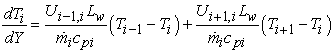(1)

Where Lw is the effective width of the channel, given by: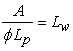(2)Download asVeiw figureFigures index
Figure 3. PHE end effect study model

If we define the following dimensionless variables: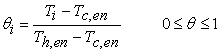(3)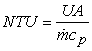(4)(5)

By developing the equation 1 and using the dimensionless variables 3, 4 and 5, we get to the following equation:(6)

Where I = 1,3,5,7,....,

It is also a modification to the proposed by Martin and Bassiouny , which includes the elongation factor .

For channel pairs where j = 2, 4, 6, 8.10...., you get the same equation by just substituting I for j.

Heat transfer coefficients and thermal effectiveness.

In order to estimate the heat transfer coefficient in plate heat exchangers the following correlation is being used [3, 4, 9, 10, 11]: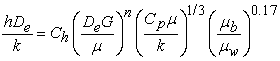(7)

Where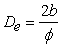is the equivalent diameter.

Ch, and n values from equation 7 depend on the flow characteristics and the chevron angle. The correlation used in this work, is reported by Kumar and colleagues [4, 9] β ≤ 30 and Re ≤ 10, Ch = 0.718 and n = 0.349. If Re> 10, Ch = 0.348 and n = 0.663, Reynolds number is calculated as:(8)

Where Gc is the mass velocity of the channel and De is the equivalent diameter of the channel: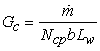(9)

The elongation factor can be calculated by the following equation: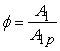(10)

Where A1 is the real effective area specified by the manufacturer, and A1P, is the projected area by the plate A1P = LPLW

If the manufacturer does not have specified the area of the heat exchanger, then the elongation factor according to Claesson J.,  is calculated as:(11)

The number of plates determines the number of pass channels of each fluid, i.e., if their number is odd or even. If the plate number is odd, then, both fluids (hot and cold) have the same number of flow channels, which is determined by the equation: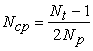(12)

If the plate number is even, then the number of channels for the hot fluid flow is determined by the equation:and the number of channels of the cold fluid is calculated by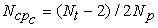where Nt is the total number of plates and Np is the number of steps.

The overall heat coefficient is calculated with the following equation, :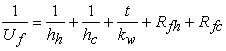(13)

The thermal effectiveness is defined in terms of the real heat transfer of hot fluid to cold fluid in a heat exchanger as a given flow under a thermodynamically possible high heat transfer. This is related to the terminal temperatures of fluids and heat capacity as[3, 4, 8, 9, 10, 16]: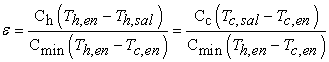(14)

In contrast, the effective temperature of hot and cold fluids is defined as: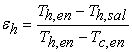(15)(16)

Equations 15 and 16 are related as:(17)

If effectiveness is based on the hot fluid, and θ is defined in terms of the outlet temperature of hot fluid then: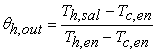(18)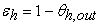(19)

Temperature correction factor

As a result of the asymmetric effect of the two end plates (end effect) of the heat exchanger, a correction factor to the logarithmic average temperature difference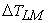must be applied, which can be defined and experimentally calculated as follows: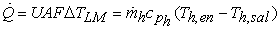(20)

Where;(21)

Once the mathematical model is solved, which gives the temperature profiles, the correction factor was calculate as:(22)

Where;(23)

### 3. Results and Discussion.

The mathematical model was analytically solved for 2, 3, 4, 5 and 6 channel flow by using a ratio of C = 0.5 to C = 1.0, varying the number of transfer units NTU. Only all the results for 5 and 6 flow channels are presented herewith.

The equations set to five channels of flow (6 plates) resulting from the arrangement shown in Figure 4 were resolved and the behavior of temperature profiles is presented in Figure 5 for a elongation factor = 1,217 and C = 0.5 and C = 1.0. As NTU increases, the temperature profiles approximate both values of C.Download asVeiw figureFigures index
Figure 4. 5-channel flow plates set upDownload asVeiw figureFigures index
Figure 5. Temperature profiles for five flow channels where ϕ= 1.217, a) NTUh,t =1.0, C= 0.5, b) NTUh,t =10, . C= 0.5, c) NTUh,t =1.0, C= 1.0, d) NTUh,t =10, C= 1.0Download asVeiw figureFigures index
Figure 6. Effectiveness of a plate heat exchanger with five flow channels, a) C= 0.5, = 1.217, b) C = -0.999, = 1.217

Figures 6 shows the thermal effectiveness and elongation factor for a plate heat exchanger with five flow channels respectively. This figure shows that when the value of the heat capacity ratio C is less than -0999 thermal efficiency is higher compared to NTUh same value, and the value tends to unity as the NTUh increases With the boundary conditions of,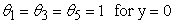andFigure 7, show the temperature profiles six flow channels C and C = -0.5 = -0999, respectively. After obtaining the correction factors to six channels of flow, we can compare the correction factors for four channels, five and six-channel flow, Figure 8.Download asVeiw figureFigures index
Figure 7. Correction factor for a plate heat exchanger with five channels of flow, a) C = -0.5, ϕ= 1.217, b) C = -0.999, ϕ= 1.217

We note that the correction factor increases in value as the number of plates increases, however, it is important to note that the correction factor is larger when you have odd channels, as in the case of channel 5 regarding four and six flow channels. From the results obtained for even channels 4 and 6 for the same value of NTUH, t, the correction factor increases, which means that the effect of the endplates is lower, which implies that increasing the transfer area heat this effect tends to decrease.Download asVeiw figureFigures index
Figure 8. Temperature profiles for six flow channels a) NTUh,t =10.0 ,C= -0.999, b) NTUh,t = 1.0, ϕ= 1.217

When comparing the correction factor of a paired channel with an odd channel for the same value of NTUH, t, the correction factor is greater for an odd channel, which implies that there is a better heat transfer and terminal effect of plates is lower in odd channels in Figure 9 shown the results for 4,5,6,7,8 and 9 channels.

According to theoretical results obtained correction factor tends rapidly towards the value of the unit to an exchanger channel with odd flow, ie a number of even plates regarding exchanger channels even flow (odd plates). Comparing the temperature correction factor of 4 and 6 flow channels (even channels), it increases in value as the number of channels, a marked tendency to approach the value of the unit as the number of plates increases, which implies that the effect of the end plates is lower as the number.

In accordance with the theoretical results for the correction factor that tends more rapidly towards the value of the unit to an exchanger with channels odd flow, ie with a number of even plates regarding exchanger channels even flow (odd plates).

Determining the deviation obtained by the factor of experimental correction versus theoretical values, show that the difference is small, but with an analysis of the standard error of the measurement, is possible determine quantitatively the accuracy of theoretical predictions for application in a larger number of flow channels.Download asVeiw figureFigures index
Figure 9. Comparison of experimental temperature correction factors for different number of channels

The standard error of estimate value is obtained by the following equation:Where Y - Y' represents the deviation and n the number of samples, which in this paper is 12.Download asVeiw figureFigures index
Figure 10. Experimental and theoretical correction factor to six flow channels and an elongation factor ϕ = 1.216

### 4. Conclusions

The solution for the proposed system consisting of a first-order differential equations representing the energy balance in a differential element taken in an even or odd channel of a heat exchanger, theoretical model yielded the temperature profiles, the thermal effectiveness and the correction factor for plate heat exchanger with different number of channels. The model considers the enlargement factor for chevron plates; to determine the correction factor of the temperature due to the effect of the endplates. Comparing the correction factor temperature for 4 and 6 flow channels (even channels) shows that it increases in value as the number of channels, this approximates the value of the unit as the number of plates increases, which implies that the effect of the end plates is lower as the number of heat exchanger plates.

### Nomenclature

A: Heat transfer area

A1: Real effective area specified by the manufacturer

A1: Projected area per plate

Ae: Effective area

cp: Heat capacity at constant pressure

De: quivalent diameter

Dh: Hydraulic diameter

Dp: Port diameter

Gc: Mass velocity in the flow channel

F: Correction factor: Total flow rate

Ncp: Number of pass channels

Np: Total steps number

Nt: Total plates number

NTUh,t: Number of hot fluid thermal units

P: Path outside the corrugated plate or spacing between the plates

Pc: Corrugation spacing

Pw: Wetted perimeter

Pr: Prandtl number

Q: Heat load required

Qf: Heat load for fouling conditions

r: Radius of the circular plate segment

Re: Reynolds Number

Rfh: Fouling factor for the hot fluid

Rfc: Fouling factor for the cold fluid

t: Plate thickness

T: Temperature

U: Overall coefficient of heat transfer

Uf: Overall coefficient of heat transfer at a fouling surface

y*: Dimensionless coordinate

y: Dimensionless coordinate

Y: Coordinate

### References

  Marriot J., Where and How To Use Plate Heat Exchangers, Chemical Engineering, April No.5, 1971, 127-133.In article  Shah, R.K., Focke, W. W., Plate Heat Exchangers and Their Design Theory, in eds. R. K. Shah, E. C. Subbarao, and R.A. Mashelkar, Heat Transfer design, Hemisphere Publishing, Washington D. C., 1988.In article  Z. H. Ayub, Plate Heat Exchanger Literature Survey and New Heat Transfer and Pressure Drop Correlations for Refrigerant Evaporators, Heat Transfer engineering, Vol. 25, No. 4, 2003, 3-16.In article View Article  Mazen M. Abu-Khader. Plate heat exchangers: Recent advances. Journal of Renewable and Sustainable Energy Reviews. Vol, 16. 2012, 1883-1891.In article View Article  Muley, A., Manglik, R. M., Experimental Study of Turbulent Flow Heat Transfer and Pressure Drop in a Plate Heat Exchanger With Chevron Plates, Transactions of The ASME., Vol. 121, February 1999, 110-117.In article  Rao Bobbili, Prabhakara., Sunden, Bengt., Das Sarit Kumar., An Experimental Investigation Of The Port To Channel Flow And Pressure Distribution Of The Smaller And Larger Plate Package Heat Exchangers, Proceedings of Fifth International Conference on Enhanced, compact and Ultra-compact Heat Exchangers: Science, Engineering and Technology . September 2005, 200-207.In article  M. K. Bassiouny, H. Martin, Temperature Distribution in a Four Channel Plate Heat Exchanger, Heat Transfer engineering, Vol. 6, No.2, 195, 1985, 58-73.In article  Manoj B. Kumbhare1 and S. D. Dawande, PERFORMANCE EVALUATION OF PLATE HEAT EXCHANGER IN LAMINAR AND TURBULENT FLOW CONDITIONS, International Journal of Chemical Sciences and Applications, Vol 4, 2013, 77-83.In article  Polley, G. T., Abu-khader, Mazen M., Compensating for End Effects in Plate and frame Heat Exchangers, Heat Transfer engineering, Vol. 26, No. 10, 2005, 3-6.In article View Article  Asadi, Masoud, “Design and Optimization of Plate and Frame Heat Exchangers,” Saarbrücken, Germany, LAP LAMBERT Academic Publishing, 2012.In article  Carla S. Fernandes, Ricardo P. Dias, João M. Nóbrega, João M. Maia. Friction factors of power-law fluids in chevron-type plate heat exchangers. Journal of Food Engineering. Vol. 89, 2008, 441-447.In article View Article  Kandlikar, S. G., Shah, R. K., Asymptotic Effectiveness-NTU Formulas for Multipass Plate Heat Exchangers, Transactions of the ASME, Vol. 111, M ay 1989, 314-323.In article View Article  Bajura, R. A., Troupe, R.A., and Morgan, J.C., Heat Transfer Design method for Plate Heat Exchangers, Chemical Engineering Progress,. Vol. 59, 1963, 57-61.In article  Tereda Fantu A., Srihari N, K. Das, and Sunden Bengt; Experimental study on Port to Channel Flor Distribution of Plate Heat Exchangers, Proceedings of Fifth International Conference on Enahanced, compact and Ultra-compact Heat Exchangers: Science, Engineering and Technology., September 2005, 208-214.In article  Rao, B. Prabhakara, Das, Sarit k., An Experimental Study on the Influence of Flow Maldistribution on the Pressure Drop Across a Plate Exchanger, Transactions of the ASME, Vol. 126, July 2004, 681-694.In article  Rao, B. Prabhakara, Das, Sarit k., Sunden, Bengt., An Experimental and Theoretical Investigation of the Effect of Flow Maldistribution on the Thermal Performance of Plate Heat Exchangers. Transactions of the ASME, Vol. 127, 2005, 332-243.In article  Claesson J., Thermal and Hydraulic Performance of Compact Brazed Plate Heat Exchangers Operating as Evaporators in domestic Heat Pumps, Doctoral thesis, Stockholm, Sweden 2004. 40-43.In article